Function Repository Resource:

# HyperbolicDistance

Give the distance between two points in hyperbolic space

Contributed by: Jan Mangaldan
 ResourceFunction["HyperbolicDistance"][u,v,"metric"] gives the distance between vectors u and v in hyperbolic space, using the specified metric.

## Details

The following metrics are supported:
 "Beltrami" Beltrami–Klein disk metric "HalfPlane" Poincaré half-plane metric "Poincare" Poincaré disk metric

## Examples

### Basic Examples (2)

Distance between two vectors on the Poincaré disk:

 In:=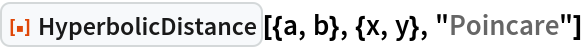Out=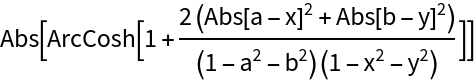Distance between numeric vectors on the Poincaré disk:

 In:=Out=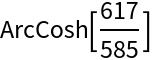### Scope (2)

Distance between two points on the Beltrami–Klein ball:

 In:=Out=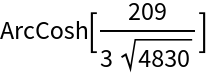Distance between two points in the Poincaré half-plane model:

 In:=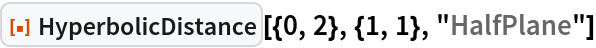Out=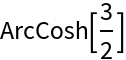### Applications (2)

Cluster data on the Poincaré ball:

 In:=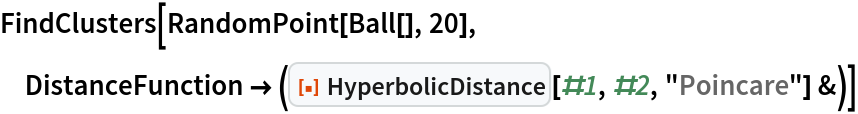Out=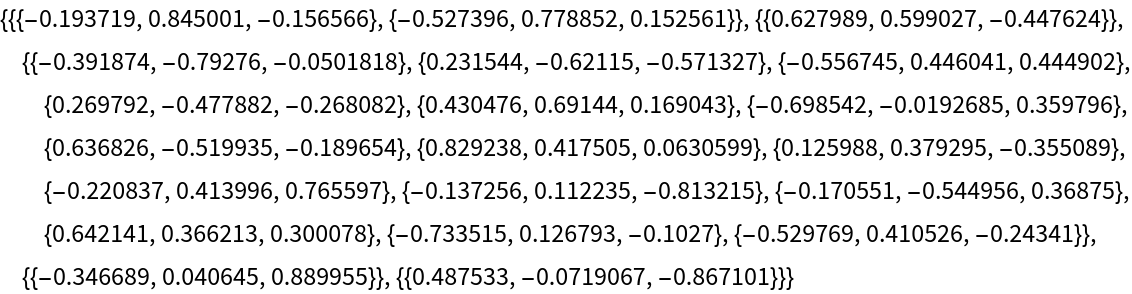Visualize the clusters:

 In:=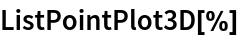Out=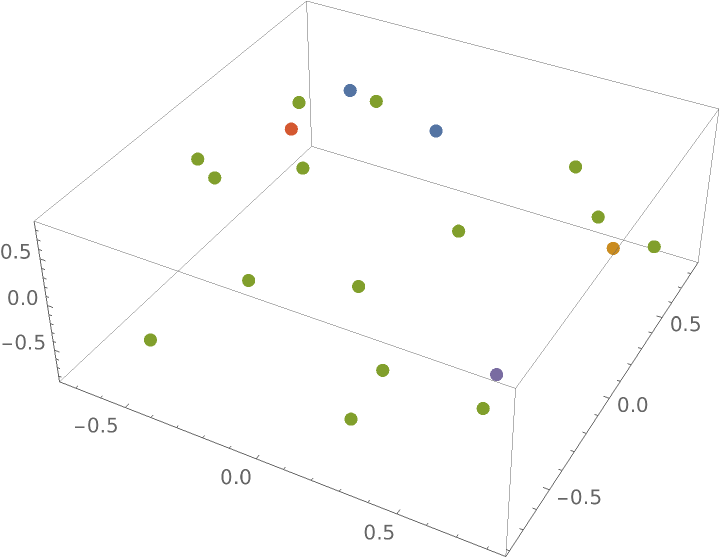### Possible Issues (1)

If at least one of the points supplied is a point at infinity, HyperbolicDistance returns Infinity:

 In:=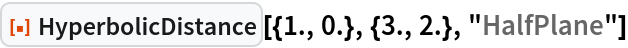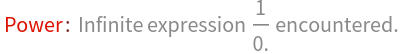Out=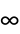### Neat Examples (1)

Visualize the Voronoi diagram of points on the Beltrami-Klein and Poincaré models:

 In:=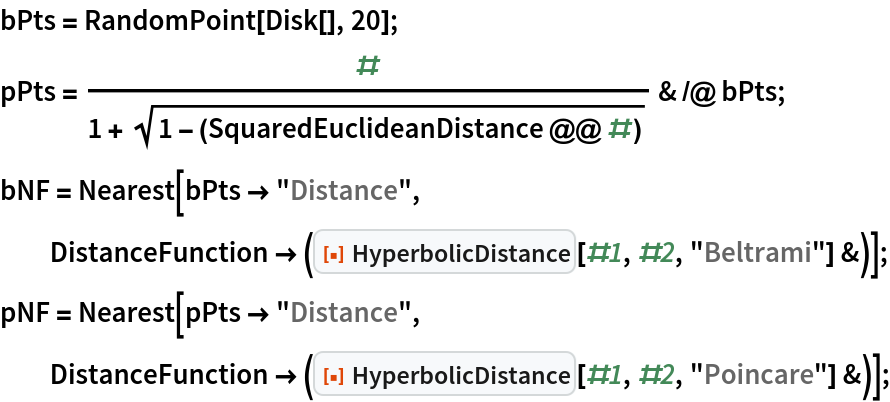In:=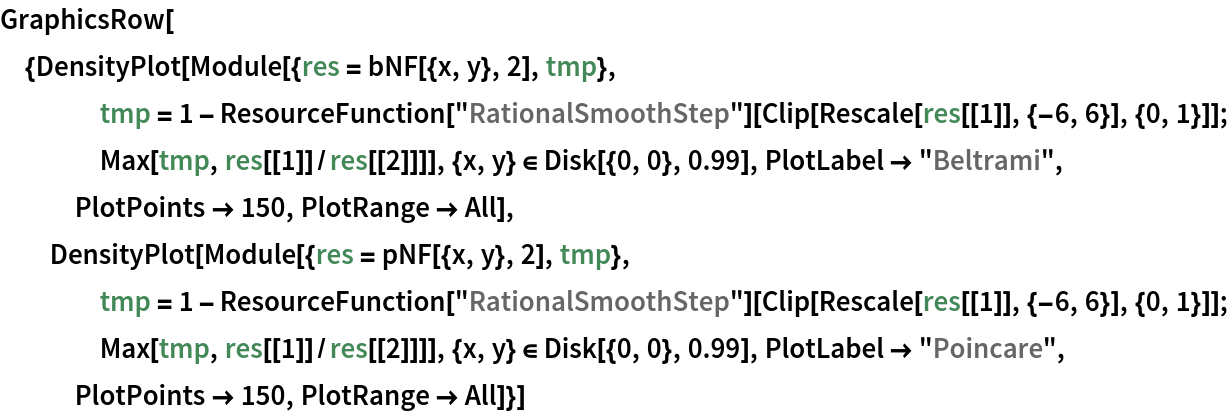Out=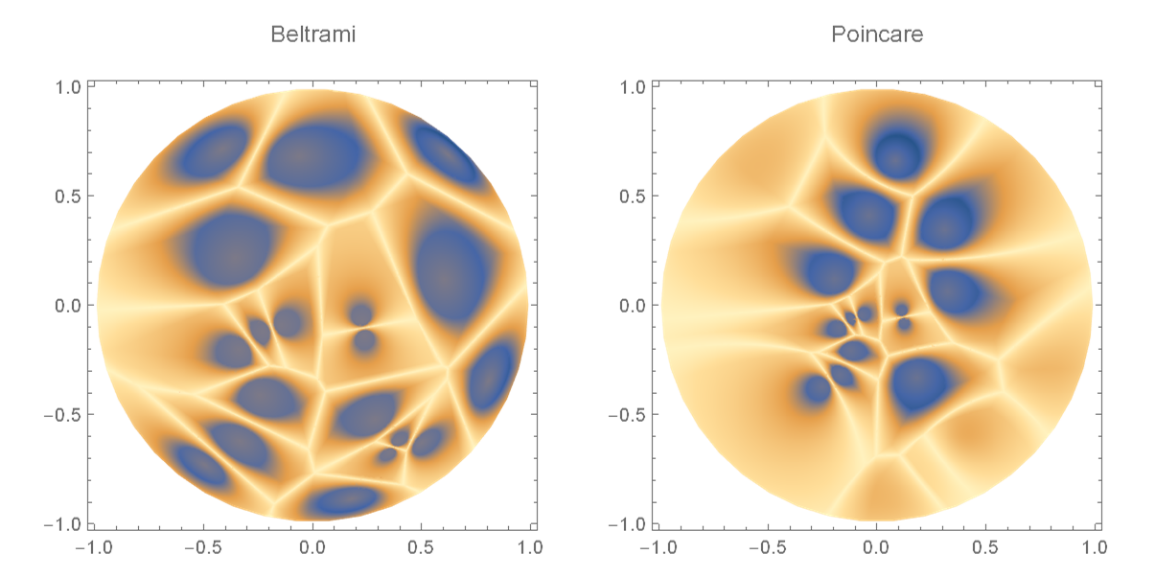## Version History

• 1.0.0 – 22 March 2021